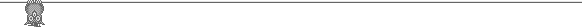## Ohkawa.cc.it-hiroshima.ac.jp9th ASU 1975 problems
1. (1) O is the circumcenter of the triangle ABC. The triangle is rotated about O to give a new triangle A'B'C'.
The lines AB and A'B' intersect at C'', BC and B'C' intersect at A'', and CA and C'A' intersect at B''. Show that
A''B''C'' is similar to ABC.
(2) O is the center of the circle through ABCD. ABCD is rotated about O to give the quadrilateral A'B'C'D'. Prove that the intersection points of corresponding sides form a parallelogram. 2. A triangle ABC has unit area. The first player chooses a point X on side AB, then the second player chooses
a point Y on side BC, and finally the first player chooses a point Z on side CA. The first player tries to arrange
for the area of XYZ to be as large as possible, the second player tries to arrange for the area to be as small as
possible. What is the optimum strategy for the first player and what is the best he can do (assuming the second
player plays optimally)?
3. What is the smallest perimeter for a convex 32-gon whose vertices are all lattice points?
4. Given a 7 x 7 square subdivided into 49 unit squares, mark the center of n unit squares, so that no four marks
form a rectangle with sides parallel to the square. What is the largest n for which this is possible? What about a
13 x 13 square?
5. Given a convex hexagon, take the midpoint of each of the six diagonals joining vertices which are separated
by a single vertex (so if the vertices are in order A, B, C, D, E, F, then the diagonals are AC, BD, CE, DF, EA,
FB). Show that the midpoints form a convex hexagon with a quarter the area of the original.
6. Show that there are 2n+1 numbers each with 2n digits, all 1 or 2, so that every two numbers differ in at least
half their digits.
7. There are finitely many polygons in the plane. Every two have a common point. Prove that there is a straight
line intersecting all the polygons.
8. a, b, c are positive reals. Show that a3 + b3 + c3 + 3abc > ab(a + b) + bc(b + c) + ca(c + a).
9. Three flies crawl along the perimeter of a triangle. At least one fly makes a complete circuit of the perimeter.
For the entire period the center of mass of the flies remains fixed. Show that it must be at the centroid of the
triangle. [You may not assume, without proof, that the flies have the same mass, or that they crawl at the same
speed, or that any fly crawls at a constant speed.]
10. The finite sequence a has each member 0, 1 or 2. A move involves replacing any two unequal members of
the sequence by a single member different from either. A series of moves results in a single number. Prove that no series of moves can terminate in a (single) different number. 11. S is a horizontal strip in the plane. n lines are drawn so that no three are collinear and every pair intersects
within the strip. A path starts at the bottom border of the strip and consists of a sequence of segments from the n
lines. The path must change line at each intersection and must always move upwards. Show that: (1) there are at
least n/2 disjoint paths; (2) there is a path of at least n segments; (3) there is a path involving not more than n/2 +
1 of the lines; and (4) there is a path that involves segments from all n lines.
12. For what n can we color the unit cubes in an n x n x n cube red or green so that every red unit cube has just
two red neighbouring cubes (sharing a face) and every green unit cube has just two green neighbouring cubes.13. p(x) is a polynomial with integral coefficients. f(n) = the sum of the (decimal) digits in the value p(n). Show
that f(n) some value m infinitely many times.
14. 20 teams each play one game with every other team. Each game results in a win or loss (no draws). k of the
teams are European. A separate trophy is awarded for the best European team on the basis of the k(k-1)/2 games
in which both teams are European. This trophy is won by a single team. The same team comes last in the overall
competition (winning fewer games than any other team). What is the largest possible value of k? If draws are
allowed and a team scores 2 for a win and 1 for a draw, what is the largest possible value of k?
15. Given real numbers a , b and positive reals c , d , let e = (a +b )/(c +d ). Let M = max
e . Show that we can find an e with 1 вүӨ i, j вүӨ n such that e = M = m . ГҷаЁҖ John Scholesjscholes@kalva.demon.co.uk1 May 2002

Source: http://ohkawa.cc.it-hiroshima.ac.jp/www.kalva.demon.co.uk.pdf/soviet/sov75.pdf

### RecuperaciГіn 2ВӘ ev.

Actividad 5 Historia de la FilosofГӯa mundo fГӯsico (cambiante, temporal, efГӯmero), solamente es posible por referencia a otra realidad (inmutable, eterna, permanente). AsГӯ, a la inicial motivaciГіn polГӯtico-moral, se aГұade una motivaciГіn epistemolГіgica que conducirГЎ el pensamiento de вҖ”вҖ”вҖ”вҖ”вҖ”вҖ”вҖ”вҖ”вҖ”вҖ”вҖ”вҖ”вҖ”вҖ”вҖ”вҖ”вҖ”вҖ”вҖ”вҖ”вҖ”вҖ”вҖ”вҖ”вҖ”вҖ”вҖ”вҖ”вҖ”вҖ”вҖ”вҖ”в

### pietdaas.nl

CHAPTER 8 Concluding remarks Abbreviations used: Co/Fe-S protein, corrinoid/iron-sulfur protein; coenzyme F , a 8-hydroxy-5-deazaflavin derivative; CoM-S-S-HTP,the heterodisulfide of coenzyme M and 7-mercaptoheptanoylthreoninephosphate; HS-CoM, coenzyme M, 2-mercaptoethanesulfonic acid;H SPT, 5,6,7,8-tetrahydrosarcinapterin; MAP, methyltransferaseactivation protein; MT , methanol:5-